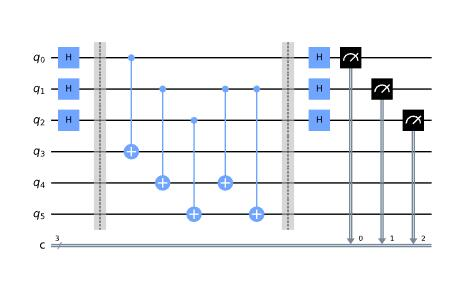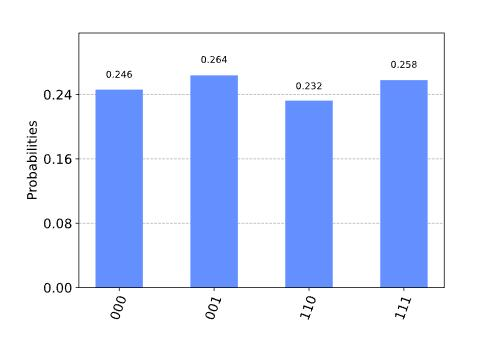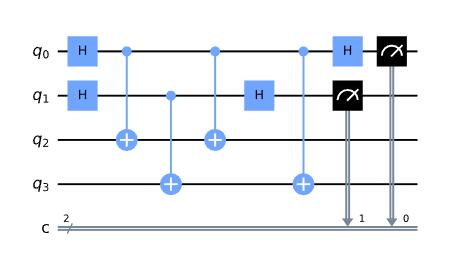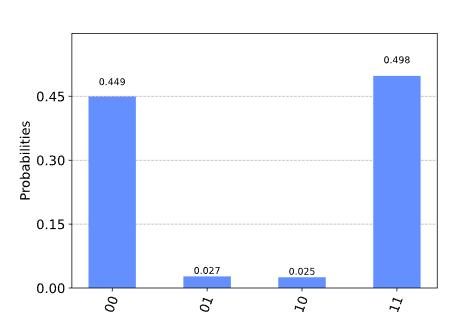# Simon算法

## 1. 简介

### 1a. Simon问题

• 一对一：对每个输入都有一个唯一的输出。一个具有4个输入的函数的例子是：
• 二对一：准确地将两个输入映射到每个唯一的输出。一个具有4个输入的函数的例子是：

\textrm{given }x_1,x_2: \quad f(x_1) = f(x_2) \\ \textrm{it is guaranteed }: \quad x_1 \oplus x_2 = b

### 量子解决方案

\ket{x} \ket{a} \rightarrow \ket{x} \ket{a \oplus f(x)}

\ket{x} \ket{0} \rightarrow \ket{x} \ket{f(x)}

1. 两个 n 量子比特输入寄存器初始化为0状态：
\ket{\psi_1} = \ket{0}^{\otimes n} \ket{0}^{\otimes n}
\ket{\psi_2} = \frac{1}{\sqrt{2^n}} \sum_{x \in \{0,1\}^{n} } \ket{x}\ket{0}^{\otimes n}
1. 应用查询函数 Q_f
\ket{\psi_3} = \frac{1}{\sqrt{2^n}} \sum_{x \in \{0,1\}^{n} } \ket{x}\ket{f(x)}
1. 测量第二个寄存器。将观察到 f(x) 的某个值。由于问题的设定，观测值 f(x) 可能对应于两种可能的输入： xy=x⊕b 。因此，第一个寄存器变为：

\ket{\psi_4} = \frac{1}{\sqrt{2}} \left( \ket{x} + \ket{y} \right)

我们省略了第二个寄存器，因为它已经被测量过了。

\ket{\psi_5} = \frac{1}{\sqrt{2^{n+1}}} \sum_{z \in \{0,1\}^{n} } \left[ (-1)^{x \cdot z} + (-1)^{y \cdot z} \right] \ket{z}
1. 测量第一个寄存器只有在以下情况下才会有输出：

(-1)^{x \cdot z} = (-1)^{y \cdot z}

这意味着：

x \cdot z = y \cdot z \\ x \cdot z = \left( x \oplus b \right) \cdot z \\ x \cdot z = x \cdot z \oplus b \cdot z \\ b \cdot z = 0 \text{ (mod 2)}

一个字符串 \ket{z} 将被测量，其与 \ket{b} 的内积等于0。因此，重复该算法大约 \ket{n} 次，我们能得到 \ket{n} 个不同的 \ket{z} 值，并可以写出以下方程组：

\begin{cases} b \cdot z_1 = 0 \\ b \cdot z_2 = 0 \\ \quad \vdots \\ b \cdot z_n = 0 \end{cases}

由此可以确定 \ket{b} ，例如通过高斯消元法。

## 2. 实例

1. 两个 2 -量子比特输入寄存器初始化为0状态：
\ket{\psi_1} = \ket{00}_1 \ket{00}_2
\ket{\psi_2} = \frac{1}{2} \left( \ket{00}_1 + \ket{01}_1 + \ket{10}_1 + \ket{11}_1 \right) \ket{00}_2
1. 对于字符串 b = 11 ，查询函数可以实现为 \text{Q}_f = CX_{1_a 2_a}CX_{1_a 2_b}CX_{1_b 2_a}CX_{1_b 2_b} (如上面的电路图所示)：

\begin{aligned} \ket{\psi_3} = \frac{1}{2} (\; &\ket{00}_1 \ket{0 \oplus 0 \oplus 0, \quad 0 \oplus 0 \oplus 0}_2 &\\ +&\ket{01}_1 \ket{0 \oplus 0 \oplus 1, \quad 0 \oplus 0 \oplus 1}_2 &\\ +&\ket{10}_1 \ket{0 \oplus 1 \oplus 0, \quad 0 \oplus 1 \oplus 0}_2 &\\ +&\ket{11}_1 \ket{0 \oplus 1 \oplus 1, \quad 0 \oplus 1 \oplus 1}_2 \;) \end{aligned}

因此：

\begin{aligned} \ket{\psi_3} = \frac{1}{2} (\; &\ket{00}_1 \ket{00}_2 &\\ +&\ket{01}_1 \ket{11}_2 &\\ +&\ket{10}_1 \ket{11}_2 &\\ +&\ket{11}_1 \ket{00}_2 \;) \end{aligned}
2. 我们测量第二个寄存器。有 50\% 的概率，我们将看到 \ket{00}_2\ket{11}_2 。为了举例，让我们假设我们看到 \ket{11}_2 。那么系统的状态是

\ket{\psi_4} = \frac{1}{\sqrt{2}} \left( \ket{01}_1 + \ket{10}_1 \right)

我们省略了第二个寄存器，因为它已经被测量过了。

\ket{\psi_5} = \frac{1}{2\sqrt{2}} \left[ \left( \ket{0} + \ket{1} \right) \otimes \left( \ket{0} - \ket{1} \right) + \left( \ket{0} - \ket{1} \right) \otimes \left( \ket{0} + \ket{1} \right) \right] \\ = \frac{1}{2\sqrt{2}} \left[ \ket{00} - \ket{01} + \ket{10} - \ket{11} + \ket{00} + \ket{01}- \ket{10} - \ket{11} \right] \\ = \frac{1}{\sqrt{2}} \left( \ket{00} - \ket{11} \right)
1. 测量第一个寄存器将以相同的概率给出 \ket{00}\ket{11}

2. 如果我们得到 \ket{11} ，那么：

b \cdot 11 = 0

这告诉我们， b≠0110 ，剩下的两个可能的解是 b=00b=11 。注意， b=00 总是我们的联立方程的一个平凡解。如果我们多次重复步骤1-6，我们将只测量 \ket{00}\ket{11} ，因为

b \cdot 11 = 0 \\ b \cdot 00 = 0

是唯一满足 b=11 的方程。我们可以通过挑选一个随机输入 (x_i) 并检查 f(x_i) = f(x_i \oplus b) 来验证 b=11 。例如：

01 \oplus b = 10 \\ f(01) = f(10) = 11

## 3. Qiskit实现

# importing Qiskit
from qiskit import IBMQ, Aer
from qiskit.providers.ibmq import least_busy
from qiskit import QuantumCircuit, transpile, assemble

# import basic plot tools
from qiskit.visualization import plot_histogram
from qiskit_textbook.tools import simon_oracle


b = '110'

n = len(b)
simon_circuit = QuantumCircuit(n*2, n)

# Apply Hadamard gates before querying the oracle
simon_circuit.h(range(n))

# Apply barrier for visual separation
simon_circuit.barrier()

simon_circuit += simon_oracle(b)

# Apply barrier for visual separation
simon_circuit.barrier()

# Apply Hadamard gates to the input register
simon_circuit.h(range(n))

# Measure qubits
simon_circuit.measure(range(n), range(n))
simon_circuit.draw()

/usr/local/anaconda3/lib/python3.7/site-packages/ipykernel_launcher.py:12: DeprecationWarning: The QuantumCircuit.__iadd__() method is being deprecated. Use the compose() (potentially with the inplace=True argument) and tensor() methods which are more flexible w.r.t circuit register compatibility.
if sys.path == '':
/usr/local/anaconda3/lib/python3.7/site-packages/qiskit/circuit/quantumcircuit.py:876: DeprecationWarning: The QuantumCircuit.extend() method is being deprecated. Use the compose() (potentially with the inplace=True argument) and tensor() methods which are more flexible w.r.t circuit register compatibility.
return self.extend(rhs)### 3a. 用模拟器进行实验

# use local simulator
aer_sim = Aer.get_backend('aer_simulator')
shots = 1024
qobj = assemble(simon_circuit, shots=shots)
results = aer_sim.run(qobj).result()
counts = results.get_counts()
plot_histogram(counts)# Calculate the dot product of the results
def bdotz(b, z):
accum = 0
for i in range(len(b)):
accum += int(b[i]) * int(z[i])
return (accum % 2)

for z in counts:
print( '{}.{} = {} (mod 2)'.format(b, z, bdotz(b,z)) )


110.000 = 0 (mod 2)
110.110 = 0 (mod 2)
110.001 = 0 (mod 2)
110.111 = 0 (mod 2)

\begin{aligned} b \cdot 001 &= 0 \\ (b_2 \cdot 0) + (b_1 \cdot 0) + (b_0 \cdot 1) & = 0 \\ (\cancel{b_2 \cdot 0}) + (\cancel{b_1 \cdot 0}) + (b_0 \cdot 1) & = 0 \\ b_0 & = 0\\ \end{aligned}

\begin{aligned} b \cdot 111 &= 0 \\ (b_2 \cdot 1) + (b_1 \cdot 1) + (\cancel{0 \cdot 1}) & = 0 \\ (b_2 \cdot 1) + (b_1 \cdot 1) & = 0 \\ \end{aligned}

b_2 = b_1 = 0, \quad b = 000

b_2 = b_1 = 1, \quad b = 110

### 3b. 用真实设备进行实验

b = '11'
n = len(b)
simon_circuit_2 = QuantumCircuit(n*2, n)

# Apply Hadamard gates before querying the oracle
simon_circuit_2.h(range(n))

# Query oracle
simon_circuit_2 += simon_oracle(b)

# Apply Hadamard gates to the input register
simon_circuit_2.h(range(n))

# Measure qubits
simon_circuit_2.measure(range(n), range(n))
simon_circuit_2.draw()# Load our saved IBMQ accounts and get the least busy backend device with less than or equal to 5 qubits
provider = IBMQ.get_provider(hub='ibm-q')
backend = least_busy(provider.backends(filters=lambda x: x.configuration().n_qubits >= n*2 and
not x.configuration().simulator and x.status().operational==True))
print("least busy backend: ", backend)

# Execute and monitor the job
from qiskit.tools.monitor import job_monitor
shots = 1024
transpiled_simon_circuit = transpile(simon_circuit_2, backend, optimization_level=3)
qobj = assemble(transpiled_simon_circuit, shots=shots)
job = backend.run(qobj)
job_monitor(job, interval=2)

least busy backend:  ibmq_mumbai
/usr/local/anaconda3/lib/python3.7/site-packages/ipykernel_launcher.py:13: DeprecationWarning: Passing a Qobj to Backend.run is deprecated and will be removed in a future release. Please pass in circuits or pulse schedules instead.
del sys.path


Job Status: job has successfully run

# Get results and plot counts
device_counts = job.result().get_counts()
plot_histogram(device_counts)# Calculate the dot product of the results
def bdotz(b, z):
accum = 0
for i in range(len(b)):
accum += int(b[i]) * int(z[i])
return (accum % 2)

print('b = ' + b)
for z in device_counts:
print( '{}.{} = {} (mod 2) ({:.1f}%)'.format(b, z, bdotz(b,z), device_counts[z]*100/shots))


b = 11
11.00 = 0 (mod 2) (44.9%)
11.01 = 1 (mod 2) (2.7%)
11.10 = 1 (mod 2) (2.5%)
11.11 = 0 (mod 2) (49.8%)

## 4. Oracle

Simon算法处理的是如何从oracle f_b 中寻找一个隐藏比特串 b \in \{0,1\}^n ，对于所有 x \in \{0,1\}^n ，满足 f_b(x) = f_b(y) 当且仅当 y = x \oplus b 。这里， \oplus 表示按位异或运算。因此，如果 b = 0\ldots 0 ，即全零比特串，则 f_b 是一个1对1(或者说，置换)函数。否则，如果 b \neq 0\ldots 0 ，则 f_b 是一个2对1函数。

• 将第一个寄存器的内容复制到第二个寄存器。
\ket{x}\ket{0} \rightarrow \ket{x}\ket{x}
• （创建1对1或2对1的映射） 如果 b 不是全零，那么有一个最小的索引 j ，使 b_j=1 。如果 x_j = 0 ，则将第二个寄存器与 b 进行异或运算。否则，不改变第二个寄存器。
\ket{x}\ket{x} \rightarrow \ket{x}\ket{x \oplus b} \text{if} ~ x_j = 0 ~ \text{for the least index j}
• （创建随机置换） 随机置换和翻转第二个寄存器的量子比特。
\ket{x}\ket{y} \rightarrow \ket{x}\ket{f_b(y)}

## 5. 问题

1. 使用Qiskit实现一个通用的Simon oracle。
2. 在模拟器和设备上，使用秘密比特串 b=1001 测试您的通用Simon oracle。结果和你预期的一样吗？说明原因。

## 6. 参考文献

1. Daniel R. Simon (1997) “On the Power of Quantum Computation” SIAM Journal on Computing, 26(5), 1474–1483, doi:10.1137/S0097539796298637
2. Guangya Cai and Daowen Qiu. Optimal separation in exact query complexities for Simon’s problem. Journal of Computer and System Sciences 97: 83-93, 2018, https://doi.org/10.1016/j.jcss.2018.05.001
import qiskit.tools.jupyter
%qiskit_version_table


### 版本信息

Qiskit Software Version
Qiskit 0.27.0
Terra 0.17.4
Aer 0.8.2
Ignis 0.6.0
Aqua 0.9.2
IBM Q Provider 0.14.0
System information
Python 3.7.7 (default, May 6 2020, 04:59:01) [Clang 4.0.1 (tags/RELEASE_401/final)]
OS Darwin
CPUs 8
Memory (Gb) 32.0

Wed Jun 16 09:23:57 2021 BST